EASY
DATA STRUCTURES AND ALGORITHMS

# How to Solve Reverse a Linked List

Written ByTom WagnerKenny Polyak

## What is the Reverse Linked List Problem?

The Reverse Linked List problem involves reversing the order of elements in a linked list, a data structure where each node is connected to the subsequent node with a pointer. The goal of this problem is to traverse the linked list while reversing the order of the pointers that link the nodes together.

### Example 1

Input: [1,2,3,4,5]
Output: [5,4,3,2,1]

Input: [1,2]
Output: [2,1]

### Example 3

Input: 
Output: 

Constraints

• The number of nodes in the list is in the range [0, 5000].
• -5000 <= Node.value <= 5000

## Solution to the Reverse a Linked List Interview Question

Before we can reverse a linked list, let’s start our approach with a concrete understanding of how a singly linked list works. After all, this interview question is an opportunity to demonstrate your familiarity with the data structure. Unlike an array, a linked list is a recursive data structure - each node points to another node - which means we don't have random access to its members. Instead, for a singly linked list we're given a head node with a next property that points to the subsequent node in the list. For a doubly linked list, we would also have a `prev` property that points to the previous node on the list. Here's an example of a linked list with two listnodes:

``````class Node:
def _init_(self, value=None):
self.value = value
self.next = None
n1 = Node(1)
n2 = Node(2)
n1.next = n2
``````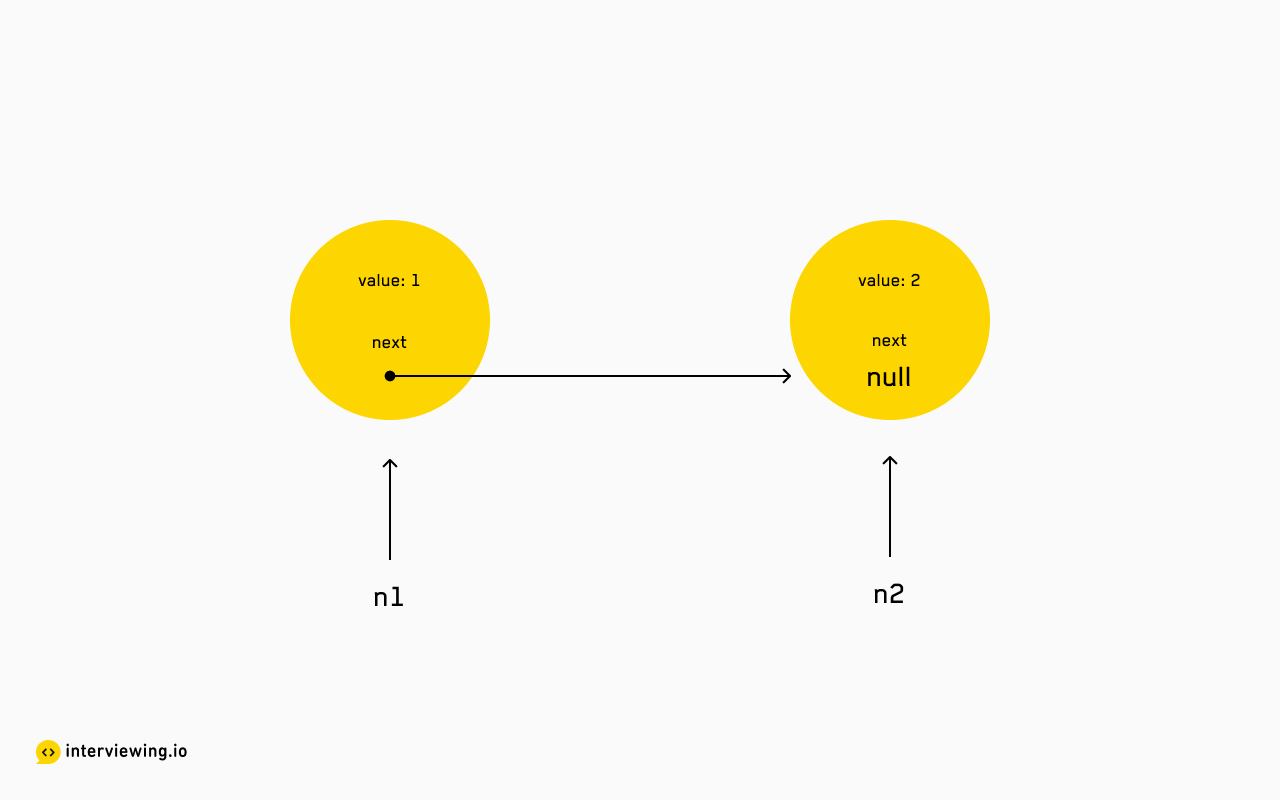In this original linked list, the first node (`n1`) points to the second node (`n2`) with the `next` property.

If this list were to be reversed, its clear that `n2` would point to `n1`. But what would `n1` point to? Recall that while `n2` does not have a child because it is at the end of the list, the node's `next` property still exists - it simply points to `null`. And while there is no node pointing to `n1`, we can imagine that its parent is `null`. So `n1` would point to `null`.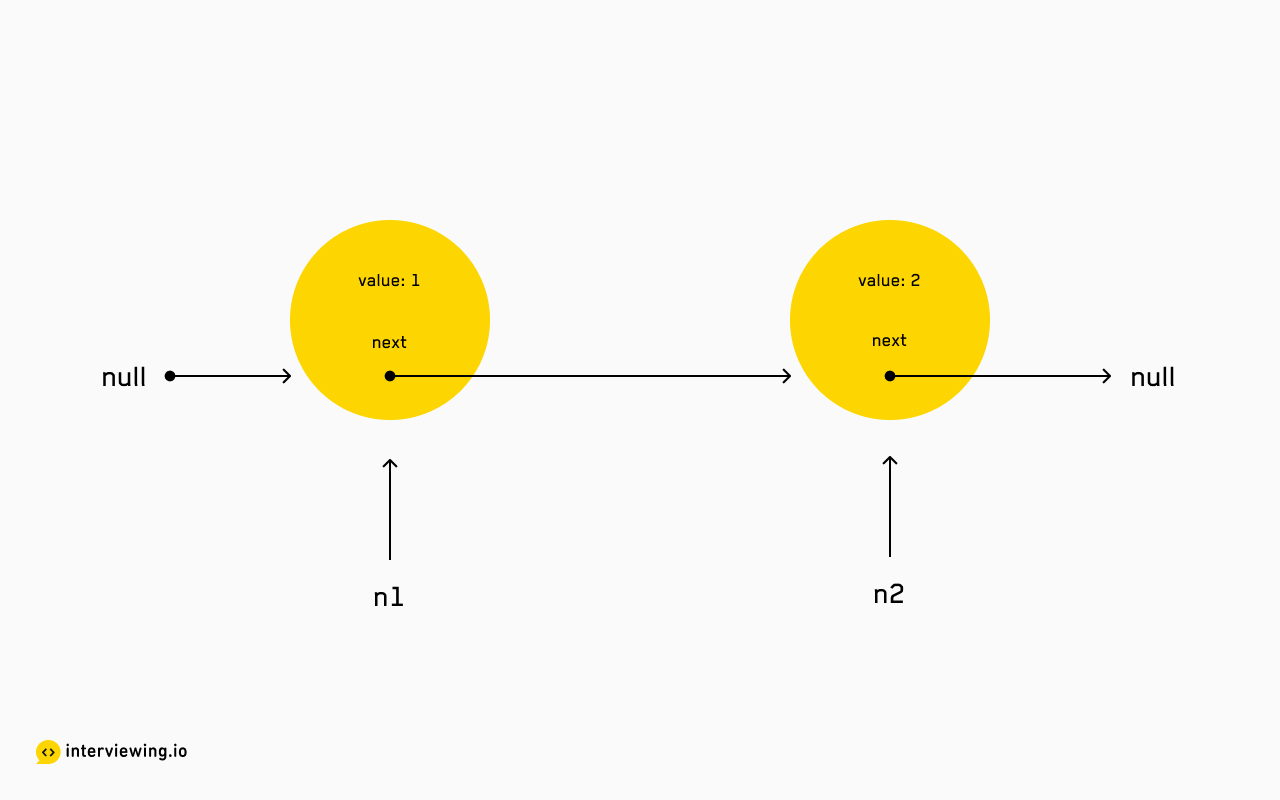We can imagine a `null` node at the head and the tail of the list.

At minimum, reversing a given linked list will require updating each node's `next` pointer to reference its parent. Since we'll need to visit each node at least once, our solution space is limited to a linear traversal.

Let's explore how we can update each linked list node in-place with a linear traversal. A linked list can be traversed both recursively and iteratively - in both approaches, we maintain a reference to the current node, its parent, and its child, and re-assign the `next` reference for each node.

### 1. Recursive Approach

Since a linked list is an inherently recursive data structure, it makes sense that we can employ a recursive approach. Let’s consider a post-order recursive traversal to reverse the list. Why "post-order"? The key here is to recurse on each subsequent node until the last node is reached, and then update the `next` pointers as each execution pops off the call stack.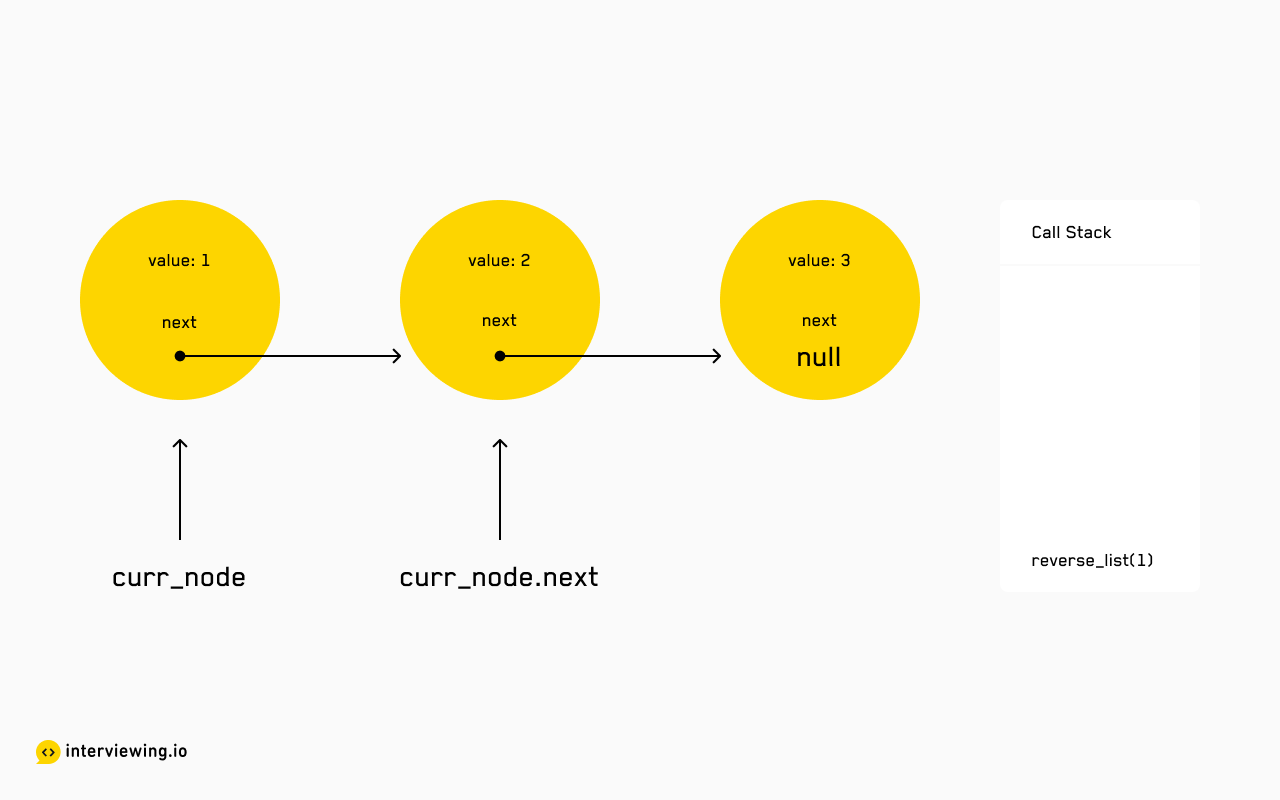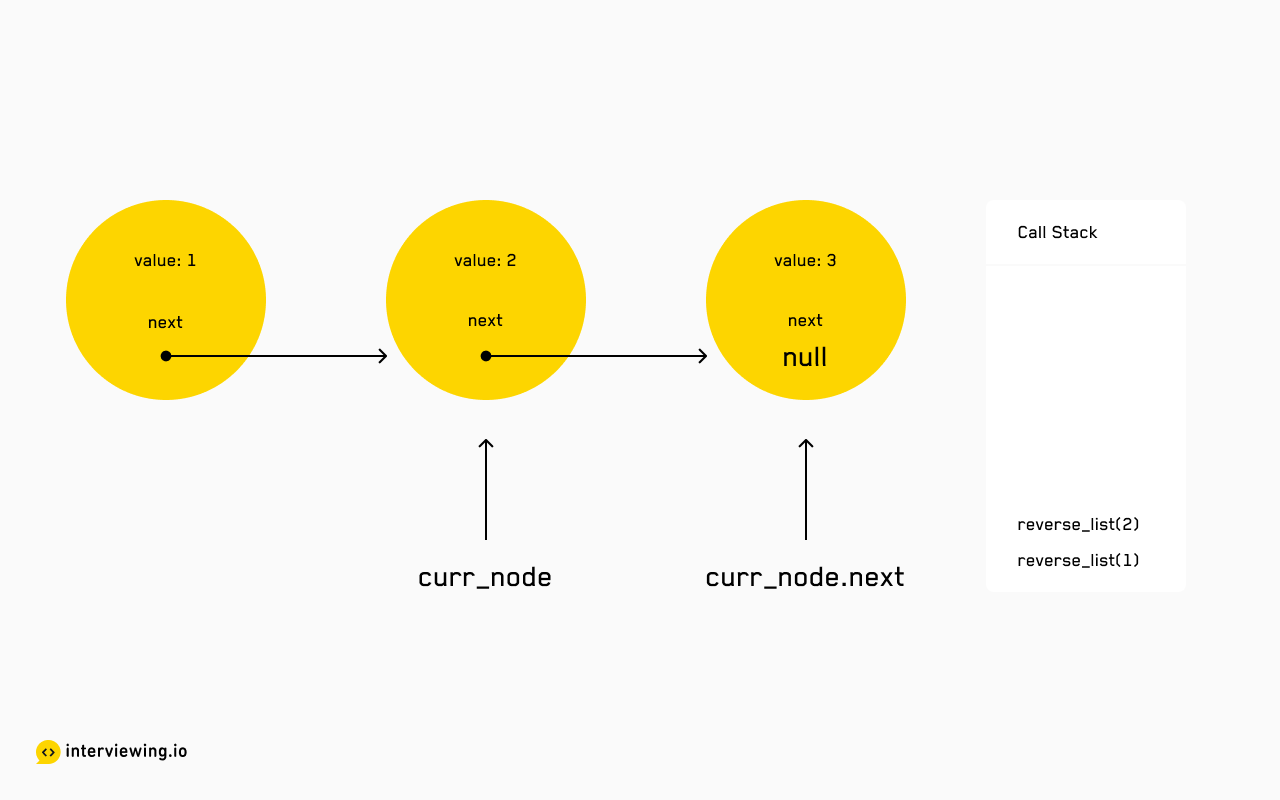Each call to the recursive function `reverse_list` is passed in a reference to the current node's child, which adds a new execution frame to the call stack.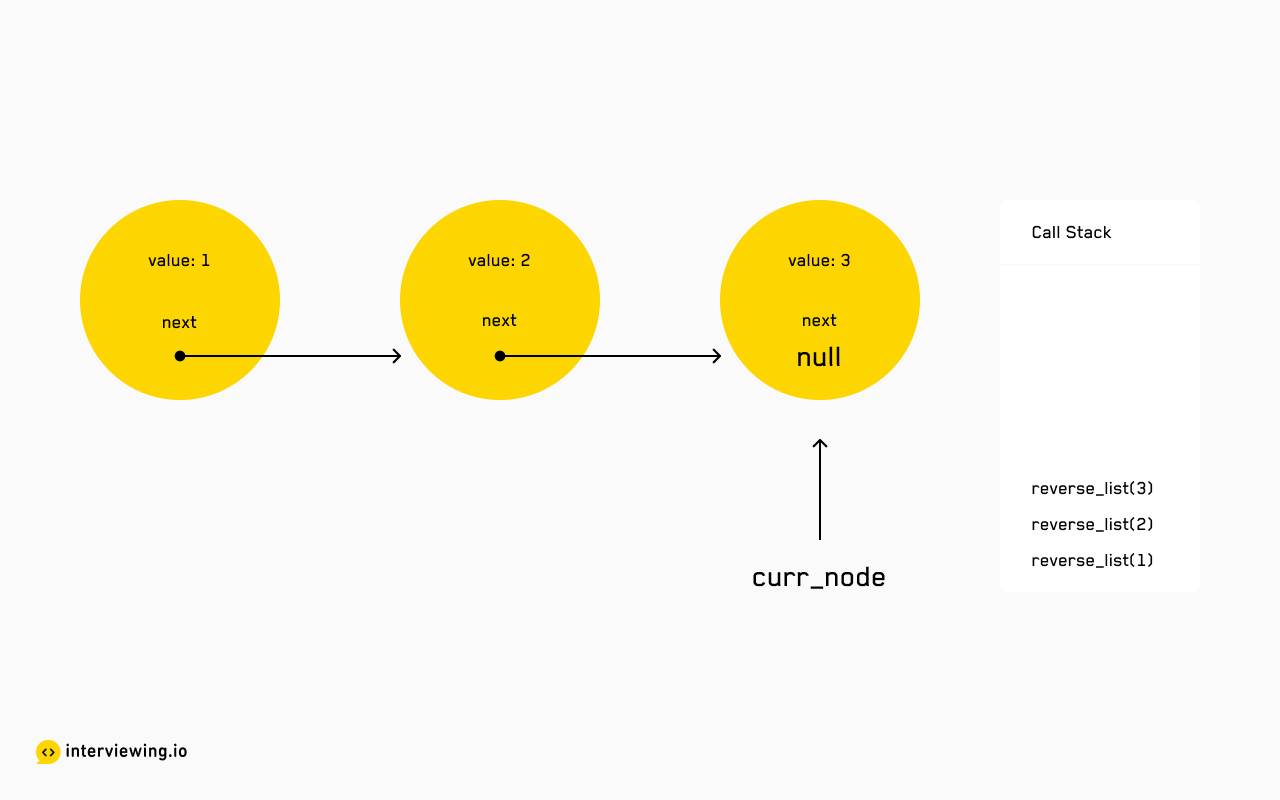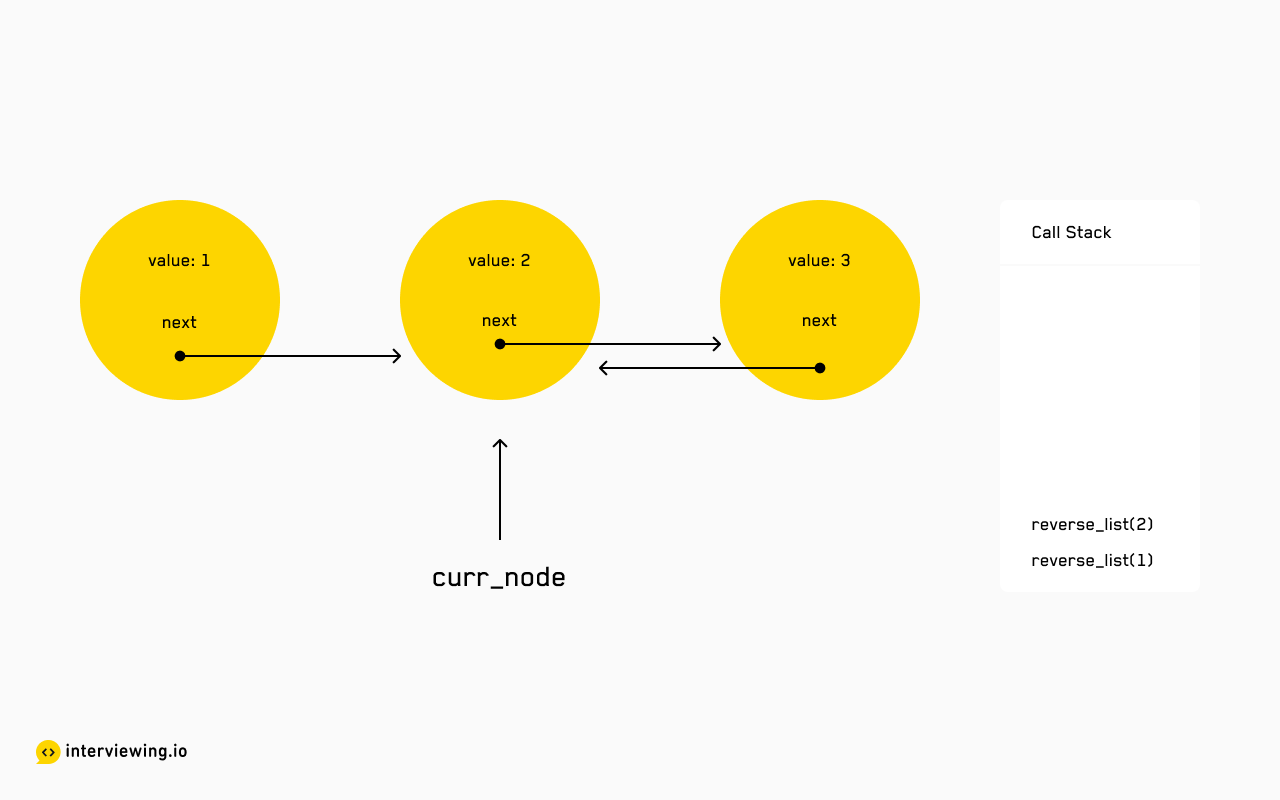Once a `null` node is reached (our base case), we begin the reassignment process. As each context is popped off the stack, we assign the current node's child's `next` pointer to the current node, effectively reversing its reference. Then return the reversed list so far.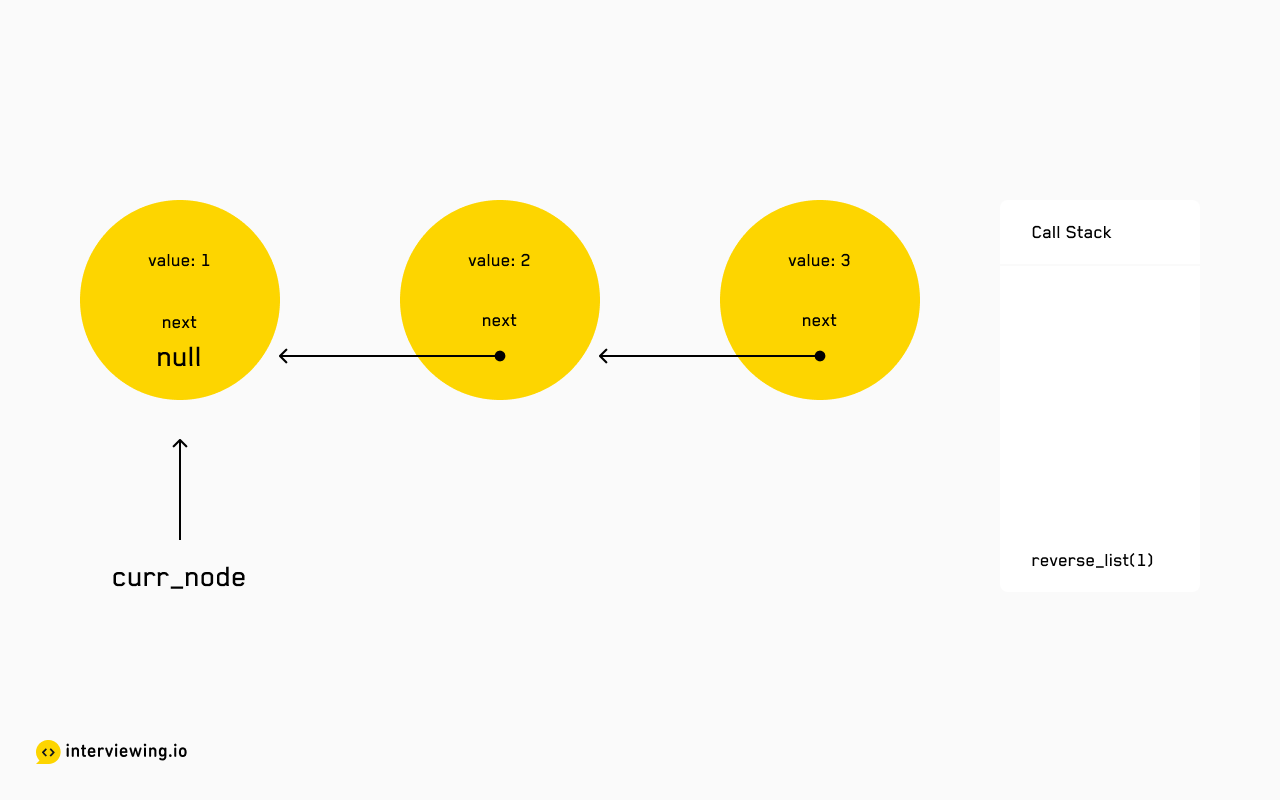We also set the current node's `next` pointer to `null` - this will be overwritten once the subsequent recursive call is resolved, except for the original head which is now the tail and therefore has no `next` node.

#### Reverse Linked List Python and JavaScript Recursive Solutions

``````class Solution:
def reverse_list(curr_node: Node) -> Node:
if not curr_node or not curr_node.next:
return curr_node
prev = self.reverse_list(curr_node.next)
curr_node.next.next = curr_node
curr_node.next = None
return prev``````

#### Time/Space Complexity

• Time Complexity: `O(n)`
• Space Complexity: `O(n)` Although we are not constructing a new linked list, recursion requires linear space on the call stack to maintain a reference to each execution context.

### 2. Iterative Approach

Reversing a linked list with an iterative approach is more space efficient than the recursive solution, and tends to be more easy to grasp. The task of any iterative traversal is managing pointers across iterations.

First, let's set up our state-of-the-world for the head (input) node.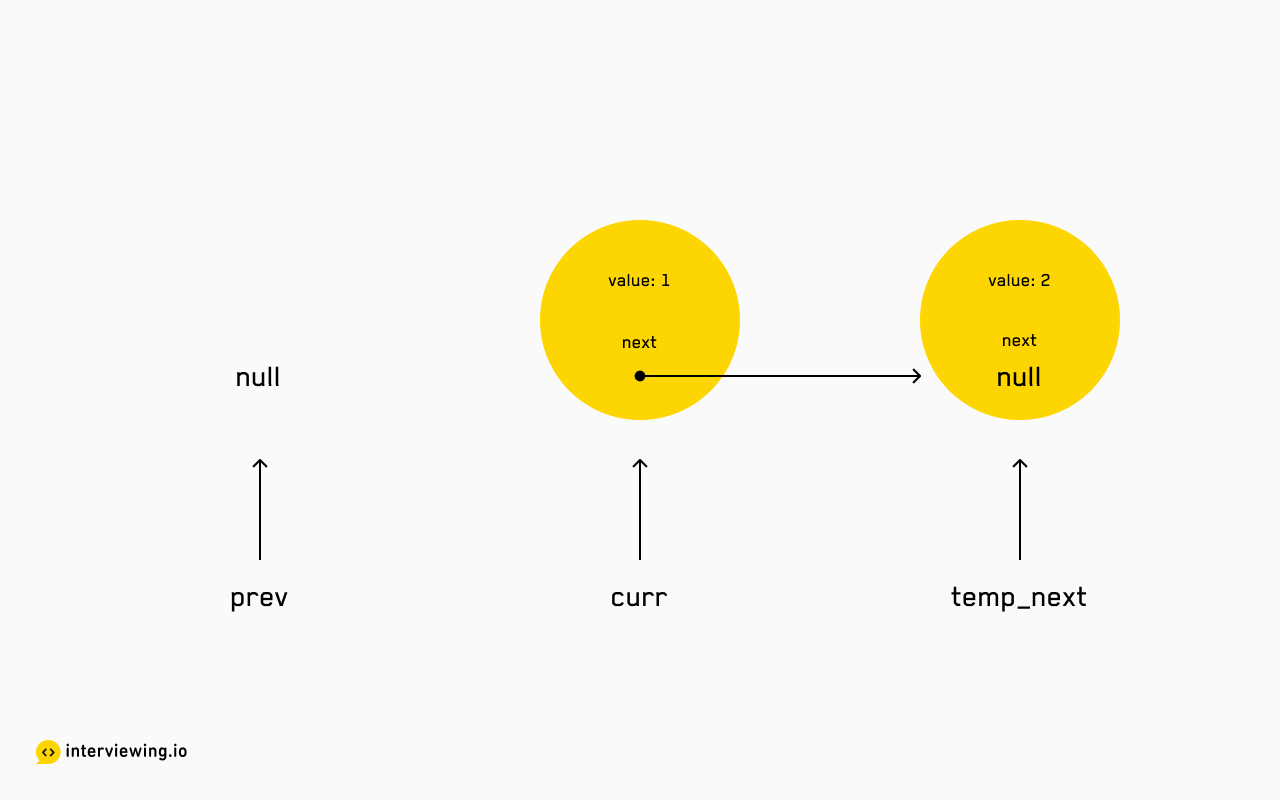`prev` points to `null` (the head has no parent), `curr` points to the current node (the head), and, if there is indeed a current node, `temp_next` points to the current node's child.At each iteration, we assign the current node's `next` pointer to the node at `prev` (reversing the reference). We then iterate forward by pointing `prev` to the current node and `curr` to the original `next` node.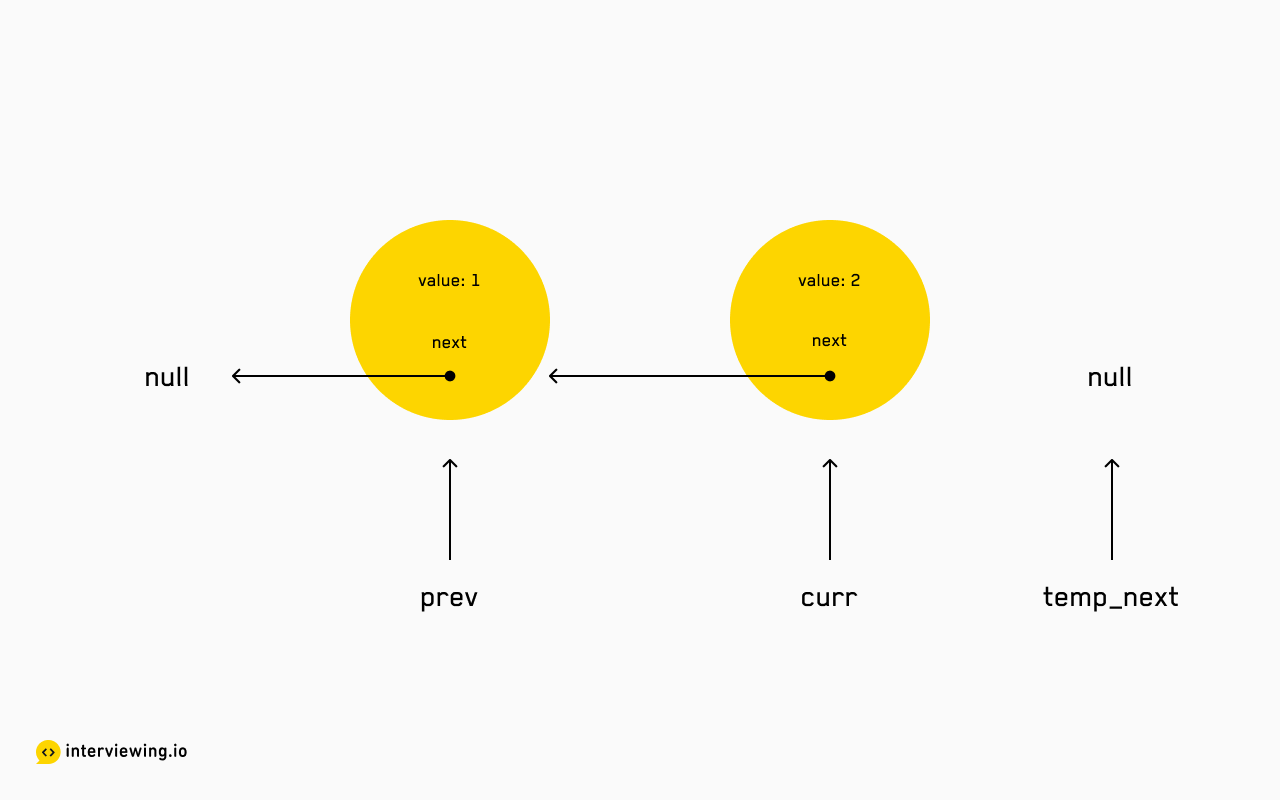We continue this process until a `null` child is reached - at which point we can return the most recent `prev` node which is our new head.

#### Reverse Linked List Python and JavaScript Solutions - Iterative

``````class Solution:
def reverse_list(self, node: Node) -> Node:
prev = None
curr = node
while curr:
temp_next = curr.next
curr.next = prev
prev = curr
curr = temp_next
return prev``````

#### Time/Space Complexity

• Time Complexity: `O(n)`
• Space Complexity: `O(1)`

## Practice the Reverse a Linked List Problem With Our AI Interviewer

Start AI Interview

### What is a linked list?

A linked list is a data structure that consists of a sequence of nodes, each of which contains two parts: a data element and a reference to the next node in the sequence. The nodes are linked together, forming a chain. Unlike an array, a linked list is a recursive data structure - each node points to another node - which means we don't have random access to its members. Instead, we're given a head node with a next property that points to the subsequent node in the list.

### Why do you reverse a linked list?

Since a singly linked list is a directional data structure, the list nodes can only be accessed in a fixed, linear order. There are a few reasons why a software engineer may need to reverse a linked list.

1. Performance optimization: if performing a search operation on a sorted linked list and you're looking for a value at the end of the list.
2. User experience: when displaying a list of messages, you may want the user to be able to reverse the sort order.
3. Traversal: if we are storing something like browser or operation history in a linked list and we want to traverse backward along the same path.

### What is the fastest way to reverse a linked list?

You can reverse a linked list with either a recursive or iterative approach. Both approaches run in linear time, `O(n)`. The recursive approach requires constant space, and the iterative approach requires `O(n)` space.

### How do you reverse a linked list without using recursion?

You can use an iterative approach! Reversing a linked list iteratively is more space efficient than recursion and tends to be more easy to grasp. The task of any iterative traversal is managing pointers across iterations. To do this, you’ll need 3 pointers: `prev`, `curr`, and `temp_next`. `prev` starts out pointing to null (the head has no parent), `curr` points to the current node (the head), and, if there is indeed a current node, `temp_next` points to the current node's child. At each iteration, we assign the current node's next pointer to the node at `prev` (reversing the reference). We then iterate forward by pointing `prev` to the current node and `curr` to the original next node. We continue this process until a null child is reached - at which point we can return the most recent `prev` node which is our new head.